# Group Short Run IX-MR Charts

Group short run individual X and moving range (IX-MR) charts can help you evaluate changes in individual measurements across multiple characteristics in a short run environment. Review the following example—an excerpt from Innovative Control Charting1—to get a sense of how a group short run IX-MR chart works.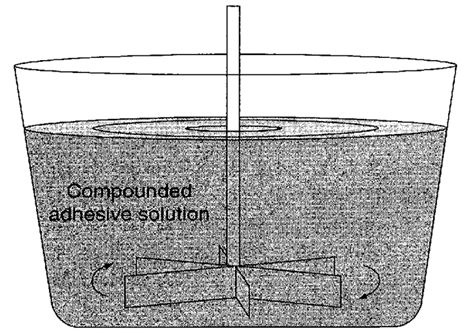Figure 1. Several parameters are monitored for each batch of compounded adhesive solution.

## Case Description

The same mixing equipment is used to mix several different types of adhesive compounds. Each compound has its own unique set of functional test requirements. In this example, three compounds are examined: compound A, B, and C. The test requirements for each are listed in Table 1.

Table 1. Test requirements for compounds A, B, and C.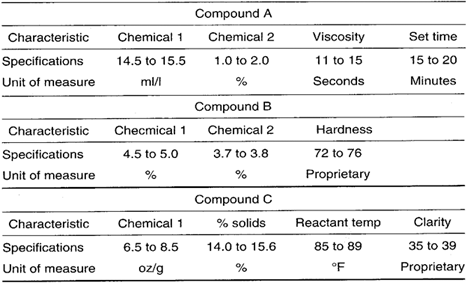## Sampling Strategy

The test characteristics, specifications, and units of measure are different for each compound, and only one measurement of each characteristic is gathered from each batch. Therefore, a group short run IX-MR chart is selected for use. Target values are established for each characteristic from each compound. The target IX values are set at the engineering nominal, but the target MR values were derived from quality assurance records.

## Target Values

Table 2. Target values for compounds A, B, and C.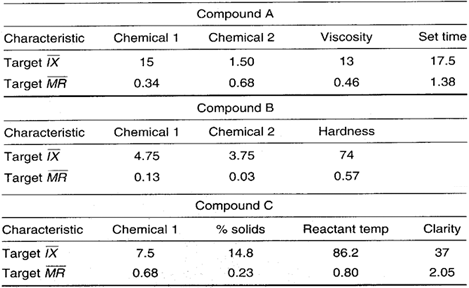## Data Collection Sheet

Table 3. Group short run IX-MR chart data collection sheet. MAX and MIN plot points are shown in bold.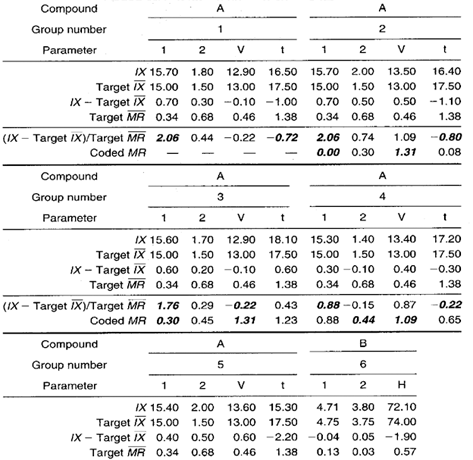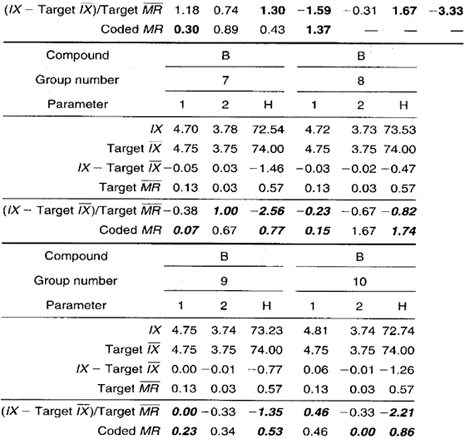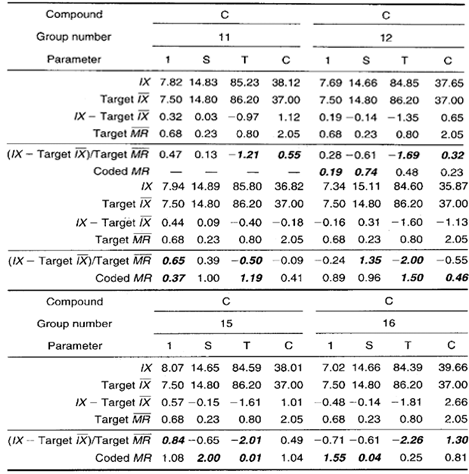## Group Short Run IX-MR Chart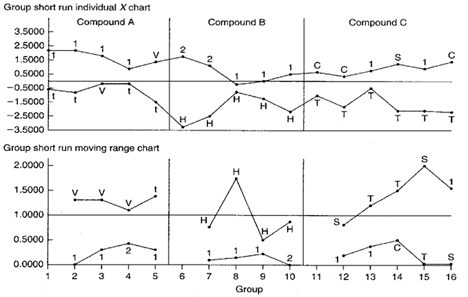Figure 2. Group short run IX-MR chart for three different compounded adhesive solutions.

## Chart Interpretation

Group short run MR chart: There is a run of four consecutive hardness (H) plot points in the MAX position from compound B. This indicates that there is significantly more variation in the hardness characteristic than others.

Also, the first 10 MIN plot points are all chemical concentrations. This indicates that the chemical concentration characteristics exhibit the lowest variability of the characteristics being evaluated regardless of the compound.

Group short run IX chart: All but one of the MAX plot points from compounds A and B represent chemical concentrations. This means that the chemical concentrations are higher on average than their targets.

All of the MIN plot points for compound B represent the hardness characteristic (H). This run indicates that the average hardness is less than its target.

The set time (t) from compound A is in the MIN position four out of five times. This may indicate that the set time is generally quicker than its target time of 17.5 minutes.

Lastly, the reactant temperature from compound C is consistently in the MIN position indicating lower than target temperatures.

## Recommendations

• The MIN plot point run of chemical concentrations on the moving range chart appears to be significant. It indicates that the standard deviations are consistently less than expected by the established target MR. Therefore, identify the cause for this improvement and update the target values.
• The MAX plot point run of chemical concentrations on the IX chart appears to be significant. The actual concentrations are consistently higher than expected by the target IX. Therefore, identify the cause(s) for these high concentrations and bring them closer to target. However, if the concentration levels were intentionally run high, the target IX values should be updated to reflect the desired concentration levels. The hardness (if) of compound B found on the group short run IX chart is consistently less than its target IX. Therefore, identify the cause and change the process to bring the hardness closer to target.
• The set time for compound A is a little faster than its target value. If this is an improvement, update the target.
• The reactant temperature (T) of compound C on the group short run IX chart is consistently less than its target value. Process personnel should attempt to do what is necessary to bring the temperature back up to target or determine if the present temperature level is desirable. If it is desirable, then the target temperature value should be updated.

## Estimating the Process Average

Estimates of the process average are calculated separately for each characteristic of each compound on the short run group chart. This is illustrated in Calculation 1 using the percent solids (S) from compound C.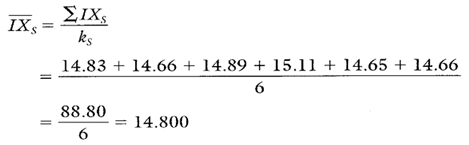Calculation 1. Estimate of the process average percent solids content(s) from compound C.

## Estimating Sigma

In estimating sigma, calculations must be performed separately for each characteristic of each compound on the group short run chart. Notice, however, that no moving ranges have been calculated—only coded MR values are shown in Table 3.

MR values should be calculated using consecutive IX values just as is done with IX-MR charts. So, in Calculations 2 and 3 and in Table 4, standard MR values have been used in calculating estimates of sigma.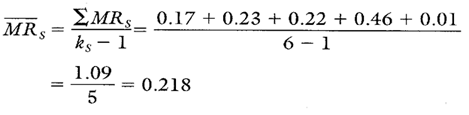Calculation 2. Average moving range for percent solids content from compound C to be used in the estimate of process standard deviation in Calculation 3.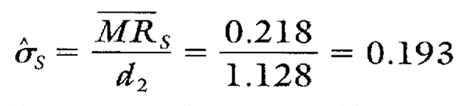Calculation 3. Estimate of the process standard deviation for percent solids content from compound C.
Note: To ensure reliable estimates of both the process average and process standard deviation, k needs to be at least 20. In this example, k is only six. Therefore, the estimates here and in Table 4 are shown only for illustration purposes.

Calculating Process Capability and Performance Ratios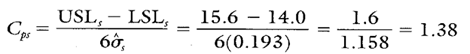Calculation 4. Cp for percent solids content from compound C.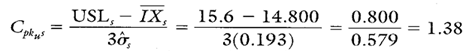Calculation 5. Cpk upper for percent solids content from compound C.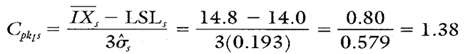Calculation 6. Cpk lower for percent solids content from compound C.

## Group Short Run IX-MR Chart Advantages

• Graphically illustrates the variation of multiple products and their characteristics simultaneously on the same chart.
• Characteristics from different parts with different means, different standard deviations, and different units of measure can all be analyzed on the same chart.
• Illustrates variation of the process and variation of the specific products.

## Group Short Run IX-MR Chart Disadvantages

• No visibility of the characteristics that fall between the MAX and MIN plot points.
• IX, MR, and estimates of sigma must be calculated separately for each characteristic on the chart.
• Analysis and recommendations can be tricky if target origins are not known.

Additional statistics and process capability and performance calculations for compound C’s chemical 1, clarity, and reactant temperature are shown in Table 4.

The largest cause for compound C’s rejections is due to reactant temperature failures. Based on the Cpkl of –0.06, more than 50 percent of the batches will fall below the lower specification. With failures this large, one of two actions ought to be considered.

1. Change the process to ensure a higher average reactant temperature. This might be a good time to perform a designed experiment to help determine what to change in the process.
2. Reexamine the need for the LSL to remain at 85°. If it can be lowered without compromising adhesive performance, change the specification and allow the average temperature to remain at its current level of 84.91°.

Table 4. Process capability and performance calculations for compound C's chemical 1, clarity, and reactant temperature.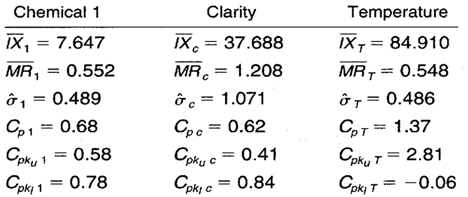When you use SPC software from InfinityQS, consuming the information provided by group short run IX-MR charts becomes faster and easier than ever. See how this type of analysis is surfaced in InfinityQS solutions.

FOOTNOTE:
1 Wise, Stephen A. and Douglas C. Fair. Innovative Control Charting: Practical SPC Solutions for Today’s Manufacturing Environment. Milwaukee, WI: ASQ Quality Press.

### Take the first step from quality to excellence

Take the Next Steps